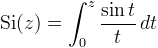Computes the sine integral.

Namespace:  Meta.Numerics.Functions
Assembly:  Meta.Numerics (in Meta.Numerics.dll) Version: 4.1.4Syntax
```public static double IntegralSi(
double x
)```

#### Parameters

x
Type: SystemDouble
The argument.

#### Return Value

Type: Double
The value of Si(x).Remarks

The sine integral is defined as:The sine integral is zero at the origin and executes a damped oscillation around π/2 as its argument increases.See Also